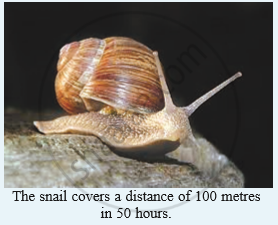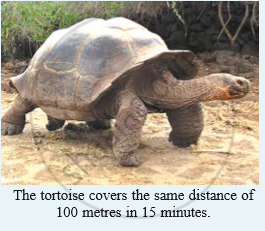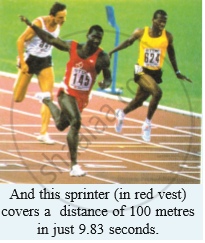Share

# A Snail Covers a Distance of 100 Metres in 50 Hours. Calculate the Average Speed of Snail in Km/H. - CBSE Class 9 - Science

ConceptMeasuring the Rate of Motion - Speed with Direction

#### Question

A snail covers a distance of 100 metres in 50 hours. Calculate the average speed of snail in km/h.#### Solution

Distance (d) = 1000 m = 0.1 km
Time (t) = 50 hr
So, we can calculate the speed as :
"Average Speed" = "Total distance travelled"/"Time"

Average speed for the entire journey :

= 0.1/50

= 0.002  km/hr

Is there an error in this question or solution?

#### Video TutorialsVIEW ALL 

Solution A Snail Covers a Distance of 100 Metres in 50 Hours. Calculate the Average Speed of Snail in Km/H. Concept: Measuring the Rate of Motion - Speed with Direction.
S